# Noughts and Crosses

Noughts and Crosses or Tic-Tac-Toe is a very simple game to play and to create with this Tutorial using Silverlight.

Step 1

Start Microsoft Visual Web Developer 2010 Express, then Select File then New Project... Select "Visual Basic" then "Silverlight Application" from Templates, select a Location if you wish, then enter a name for the Project and then click OK, see below: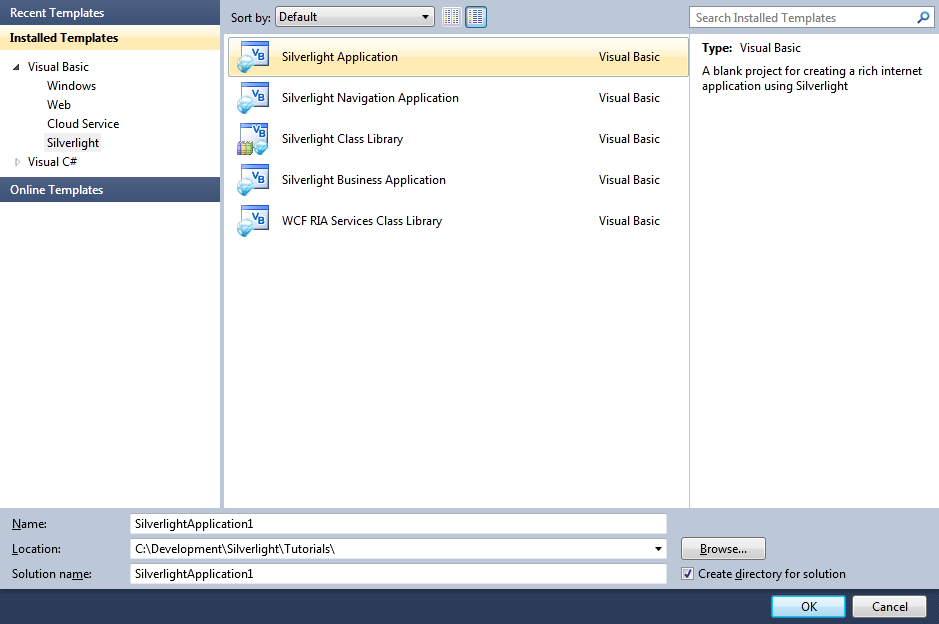Step 2

New Silverlight Application window should appear, uncheck the box "Host the Silverlight Application in a new Web site" and then select the required Silverlight Version, see below: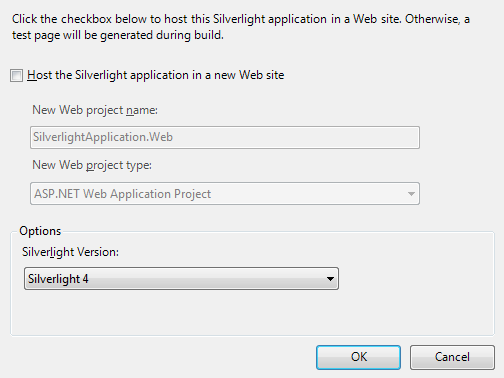Step 3

A Blank Page named MainPage.xaml should then appear, see below:Step 4

Then from the All Silverlight Controls section in the Toolbox select the Canvas control: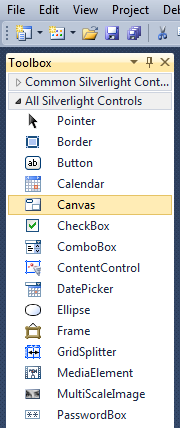Step 5

Draw a Canvas that fill the whole Page or in the XAML Pane between the "<Grid>" and "</Grid>" lines type the following XAML:

```<Canvas Height="300" Width="400" HorizontalAlignment="Left" VerticalAlignment="Top" Name="Page">
</Canvas>
```

See below: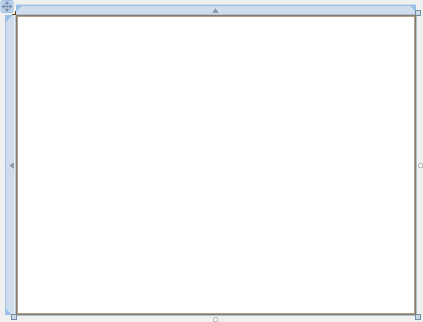Step 6

Then from the Common Silverlight Controls section in the Toolbox select the Grid control: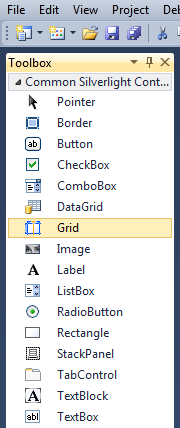Step 7

Draw a Grid on the Canvas by dragging a Grid from the Toolbox onto the Canvas then in the XAML Pane inbetween the "<Canvas>" and "</Canvas>" tags change the "<Grid> line to the following:

```<Grid Canvas.Left="75" Canvas.Top="0" Height="250" Width="250" Name="Display"/>
```

See below:Step 8

Then from the Common Silverlight Controls section in the Toolbox select the Button control: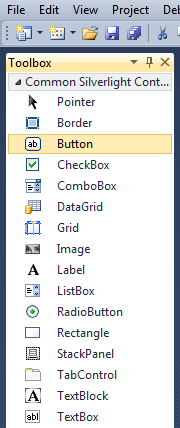Step 9

Draw a Button on the Canvas by dragging the Button from the Toolbox onto the Canvas, then in the XAML Pane below the "<StackPanel>" tag change the "<Button>" line to the following:

```<Button Canvas.Left="163" Canvas.Top="263" Height="23" Width="75" Name="New" Content="New"/>
```

See below:Step 10

Right Click on the Page or the entry for "MainPage.xaml" in Solution Explorer and choose the "View Code" option. In the Code View below the "Inherits UserControl" line type the following:

```Private _hasWon As Boolean = False
Private _piece As String = ""
Private _nought As String = "O"
Private _cross As String = "X"
Private _board(3, 3) As String
```

See Below: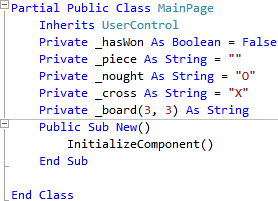Step 11

While still in the Code View for MainPage.xaml, below the "End Sub" for "Public Sub New()" Constructor type the following Functions:

```Private Function Winner() As Boolean
Return (_board(0, 0) = _piece And _board(0, 1) = _piece And _board(0, 2) = _piece) Or _
(_board(1, 0) = _piece And _board(1, 1) = _piece And _board(1, 2) = _piece) Or _
(_board(2, 0) = _piece And _board(2, 1) = _piece And _board(2, 2) = _piece) Or _
(_board(0, 0) = _piece And _board(1, 0) = _piece And _board(2, 0) = _piece) Or _
(_board(0, 1) = _piece And _board(1, 1) = _piece And _board(2, 1) = _piece) Or _
(_board(0, 2) = _piece And _board(1, 2) = _piece And _board(2, 2) = _piece) Or _
(_board(0, 0) = _piece And _board(1, 1) = _piece And _board(2, 2) = _piece) Or _
(_board(0, 2) = _piece And _board(1, 1) = _piece And _board(2, 0) = _piece)
End Function

Private Function Drawn() As Boolean
Return _board(0, 0) <> "" And _board(0, 1) <> "" And _board(0, 2) <> "" _
And _board(1, 0) <> "" And _board(1, 1) <> "" And _board(1, 2) <> "" _
And _board(2, 0) <> "" And _board(2, 1) <> "" And _board(2, 2) <> ""
End Function
```

See Below: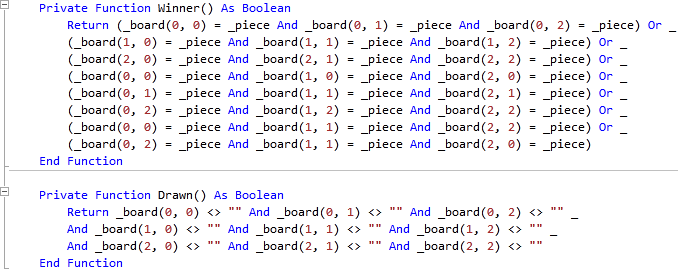Step 12

While still in the Code View for MainPage.xaml, below "End Function" for "Private Function Drawn()" Function, type the following Sub:

```Private Function GetPiece() As Path
If _piece = _cross Then ' Draw X
Dim _lines As New Path
Dim _line1, _line2 As New LineGeometry
Dim _linegroup As New GeometryGroup
_line1.StartPoint = New Point(0, 0)
_line1.EndPoint = New Point(50, 50)
_line2.StartPoint = New Point(50, 0)
_line2.EndPoint = New Point(0, 50)
_lines.Data = _linegroup
_lines.Stroke = New SolidColorBrush(Colors.Red)
_lines.StrokeThickness = 4.0
_lines.Margin = New Thickness(5)
Return _lines
Else ' Draw O
Dim _ellipse As New EllipseGeometry
Dim _circle As New Path
_ellipse.Center = New Point(25, 25)
_circle.Data = _ellipse
_circle.Stroke = New SolidColorBrush(Colors.Blue)
_circle.StrokeThickness = 4.0
_circle.Margin = New Thickness(5)
Return _circle
End If
End Function
```

See Below: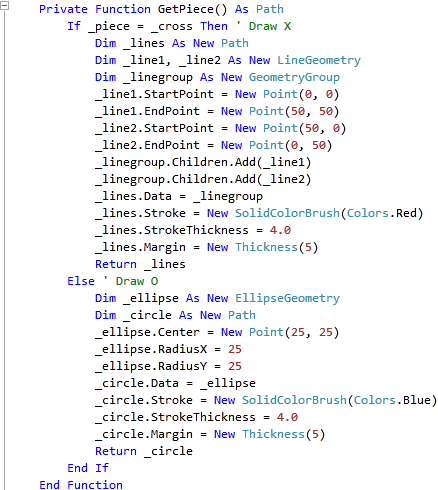Step 13

While still in the Code View for MainPage.xaml, below the "End Function" for "Private Function GetPiece()" type the following Sub:

```Private Sub Button_Click(ByVal sender As System.Object, _
ByVal e As System.Windows.RoutedEventArgs)
If Not _hasWon Then
Dim _btn As New Button
_btn = CType(sender, Button)
If _btn.Content Is Nothing Then
_btn.Content = GetPiece()
_board(_btn.GetValue(Grid.RowProperty), _
_btn.GetValue(Grid.ColumnProperty)) = _piece
End If
If Winner() Then
_hasWon = True
MessageBox.Show(_piece & " wins!", "Noughts and Crosses", MessageBoxButton.OK)
ElseIf Drawn() Then
MessageBox.Show("Draw!", "Noughts and Crosses", MessageBoxButton.OK)
Else
_piece = IIf(_piece = _cross, _nought, _cross) ' Swap Players
End If
Else
MessageBox.Show("Game Over!", "Noughts and Crosses", MessageBoxButton.OK)
End If
End Sub
```

See Below: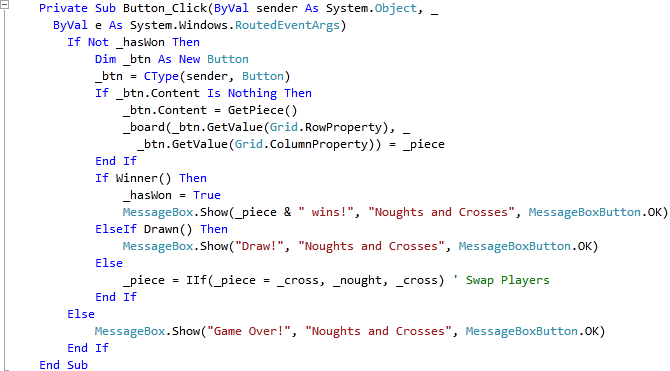Step 14

While still in the Code View for MainPage.xaml, below the "End Sub" for "Private Sub Button_Click(...)" type the following Subs:

```Private Sub Add(ByRef Grid As Grid, ByRef Row As Integer, ByRef Column As Integer)
Dim _btn As New Button
_btn.Content = Nothing
_btn.Margin = New Thickness(5)
_btn.SetValue(Grid.ColumnProperty, Column)
_btn.SetValue(Grid.RowProperty, Row)
End Sub

Private Sub Layout(ByRef Grid As Grid)
Grid.Children.Clear()
Grid.ColumnDefinitions.Clear()
Grid.RowDefinitions.Clear()
For Index As Integer = 0 To 2 ' Setup 3x3 Grid
Next
Add(Grid, 0, 0) ' Top Left
Add(Grid, 0, 1) ' Top Middle
Add(Grid, 0, 2) ' Top Right
Add(Grid, 1, 0) ' Middle Left
Add(Grid, 1, 2) ' Middle Right
Add(Grid, 2, 0) ' Bottom Left
Add(Grid, 2, 1) ' Bottom Middle
Add(Grid, 2, 2) ' Bottom Right
End Sub
```

See Below:Step 15

Return to the Designer View, by selecting the "MainPage.xaml" Tab, or Right Click on the Page or the Entry for "MainPage.xaml" in Solution Explorer and choose the "View Designer" option.
Double Click on the "New" Button Control and type in the New_Click Sub:

```Layout(Display)
_board(0, 0) = "" ' Top Left
_board(0, 1) = "" ' Top Middle
_board(0, 2) = "" ' Top Right
_board(1, 0) = "" ' Middle Left
_board(1, 1) = "" ' Centre
_board(1, 2) = "" ' Middle Right
_board(2, 0) = "" ' Bottom Left
_board(2, 1) = "" ' Bottom Middle
_board(2, 2) = "" ' Bottom Right
_hasWon = False
If MessageBox.Show(_cross & " to go first?", "Noughts and Crosses", _
MessageBoxButton.OKCancel) = MessageBoxResult.OK Then
_piece = _cross
Else
_piece = _nought
End If
```

See Below: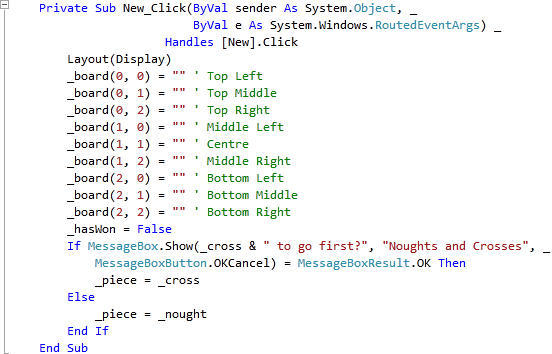Step 16

Save the Project as you have now finished the Silverlight application. Select Debug then Start Debugging or click on Start Debugging:After you do, the following will appear in a new Web Browser window: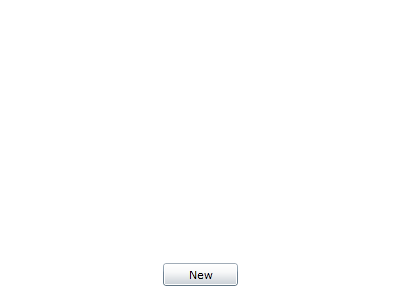Step 17

Click the New Button, then it will ask "X to go first?", choose either OK or Cancel for "O" to go First, and the following will appear: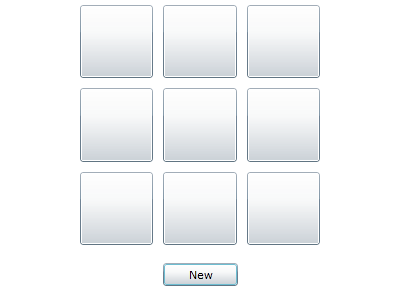Step 18

Click on the Buttons to place a "O" or an "X", continue until you Win, Lose or Draw, see below: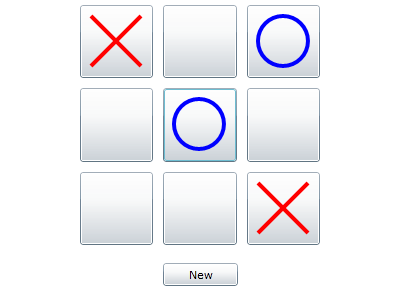Step 19

Close the Browser window by clicking on the Close Button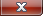on the top right of the Web Browser to Stop the application.

This is a very simple game using Silverlight, try adding some more advanced features such as a Computer-based Player 2 using Random Numbers or just change the colours, make it your own!